Abstract: 数字图像处理：第45天
Keywords: Sobel算子,Scharr算子,Prewitt算子

# 灰度图像-图像分割 Sobel算子，Prewitt算子和Scharr算子平滑能力比较

## 数学原理

$$Re=\frac{1}{n}\sum_{i=1}^nd^2_i$$

## 代码

Matlab写程序写的不多，所以将就看。

#实验结果

$\frac{1}{4}\times[1,2,1]$，$\frac{1}{3}\times[1,1,1]$，$\frac{1}{16}\times[3,10,3]$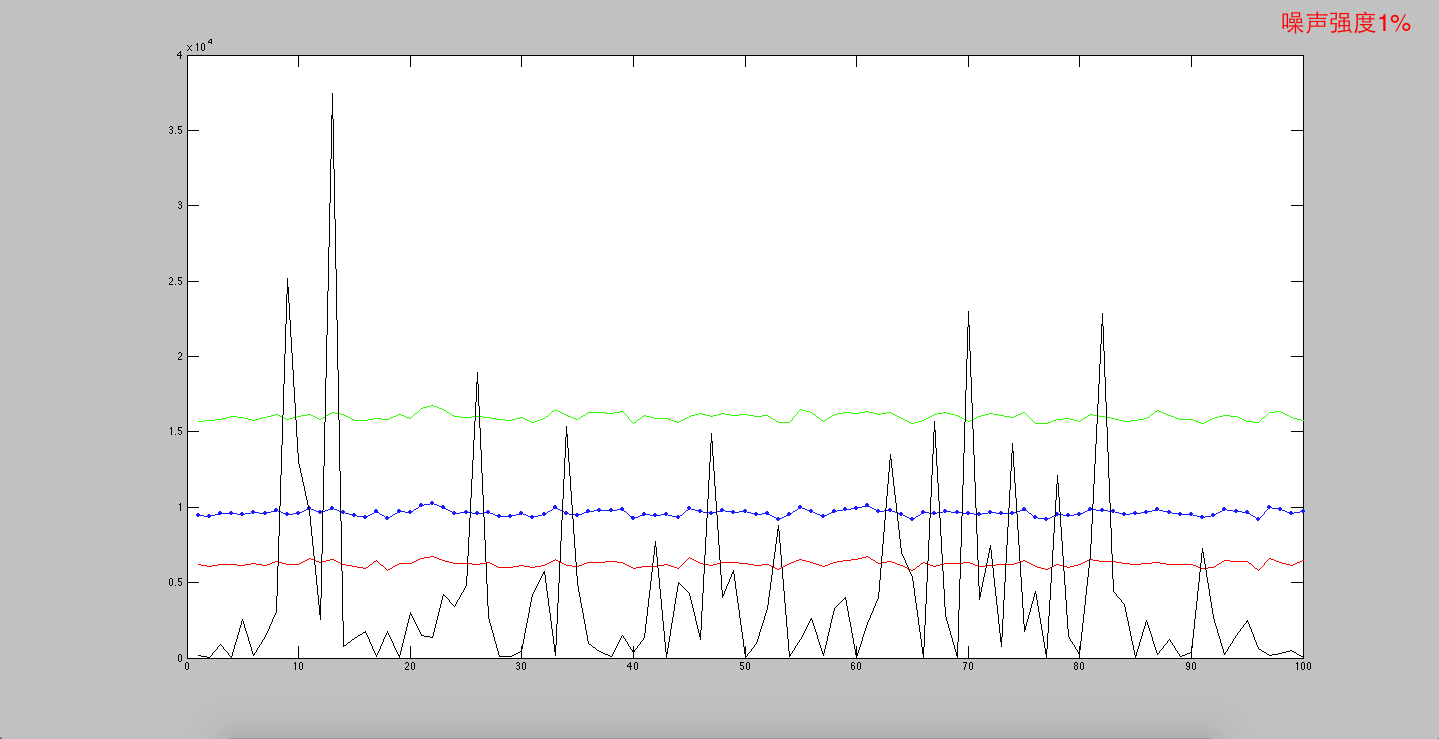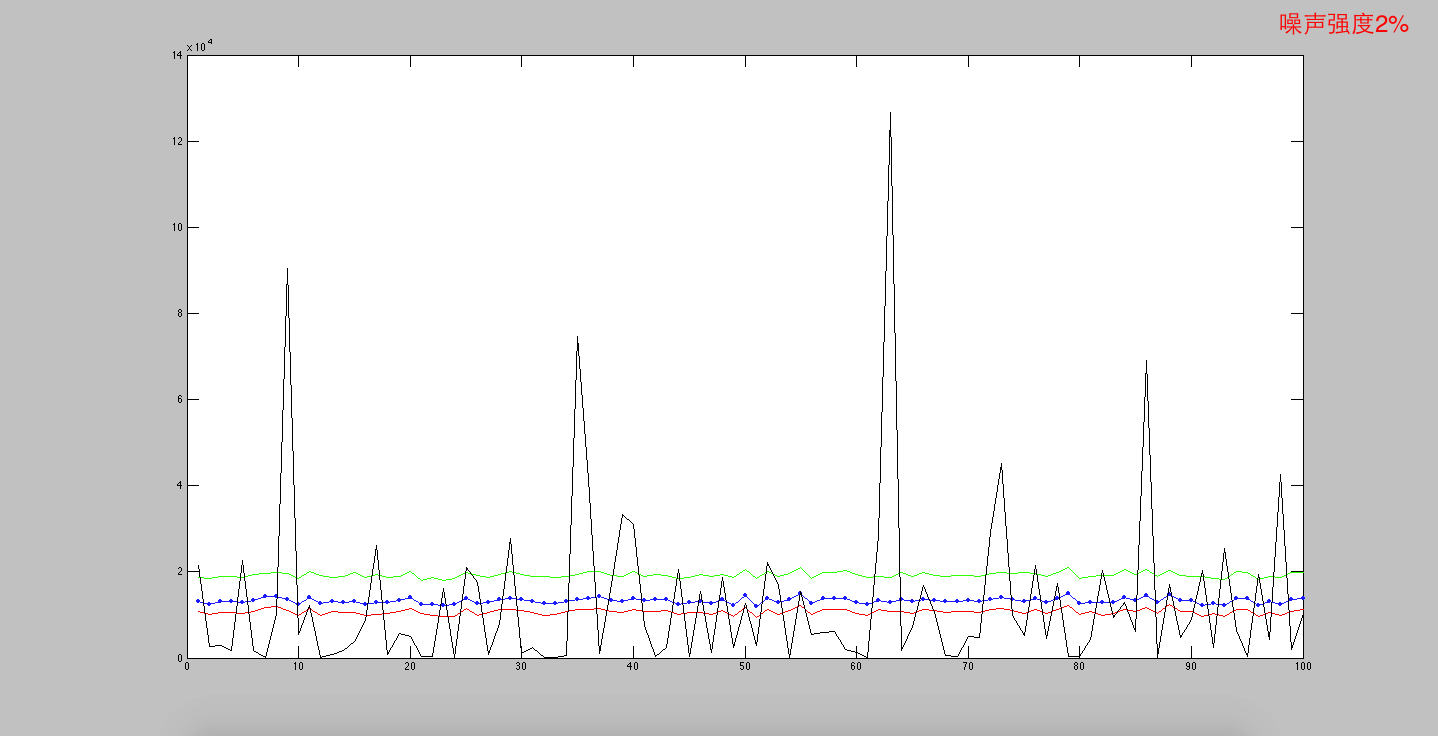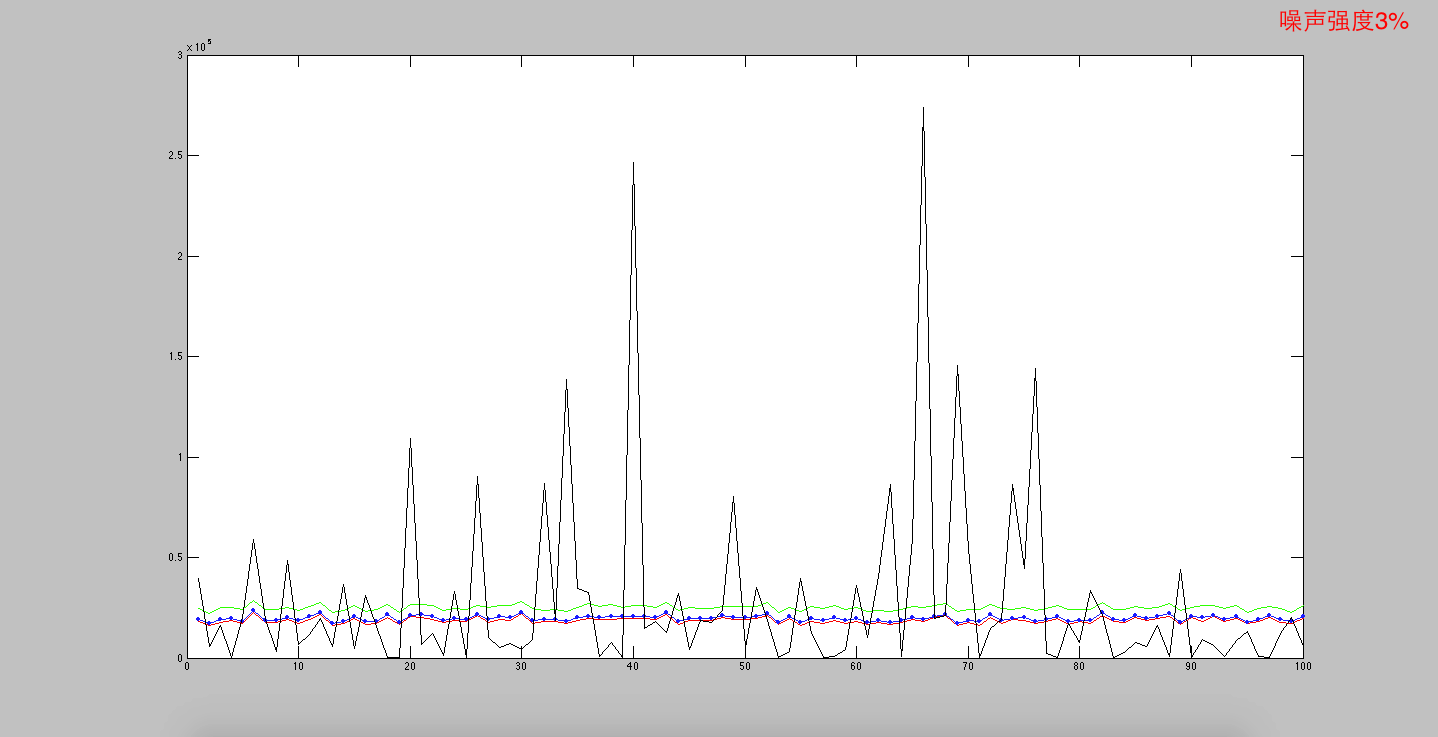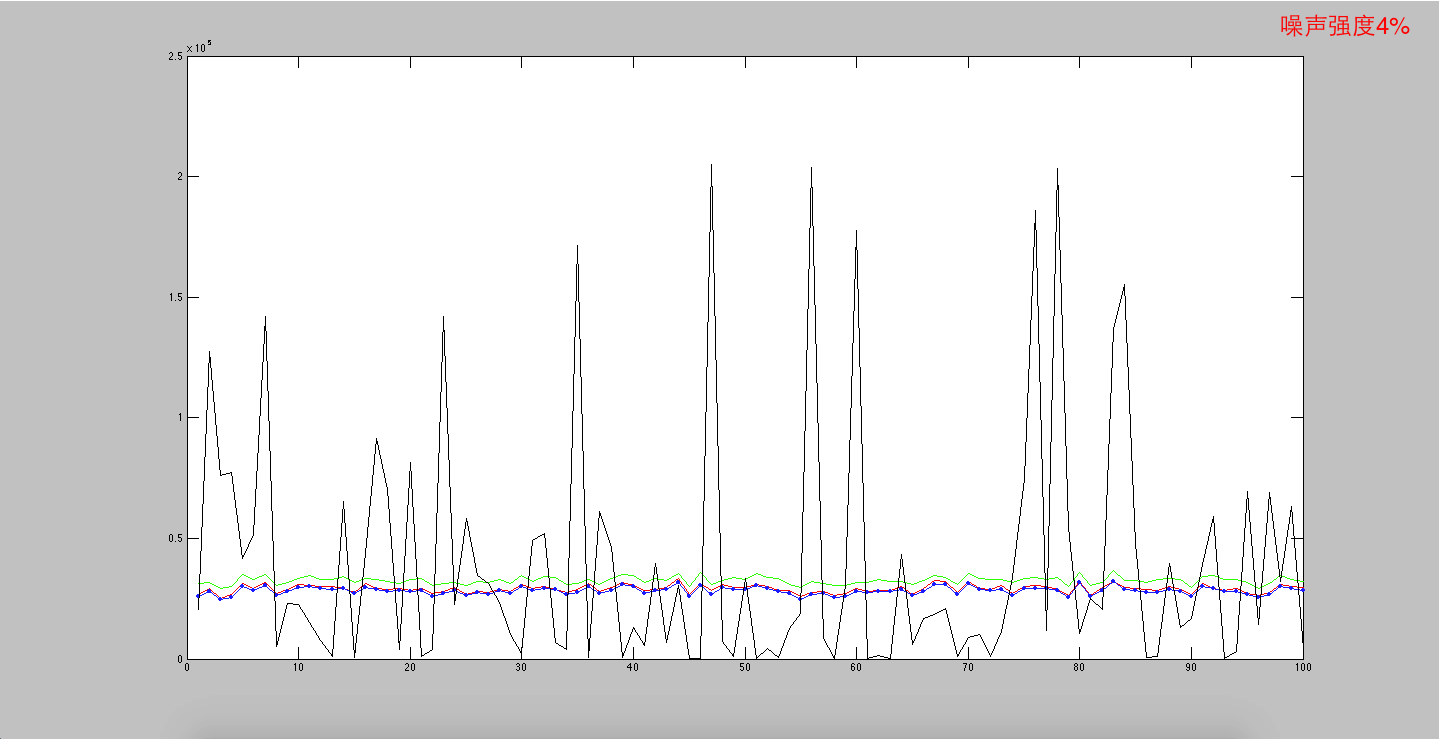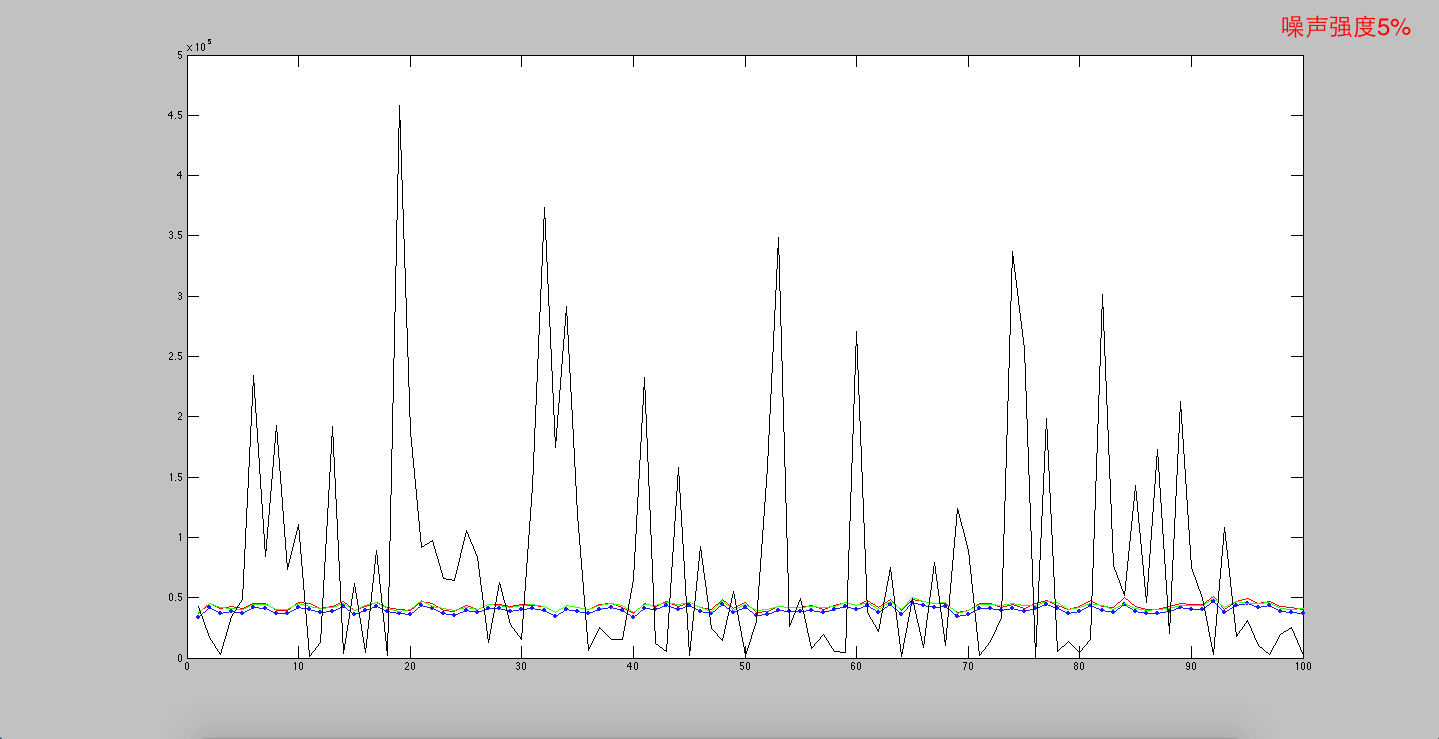0%